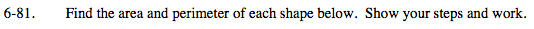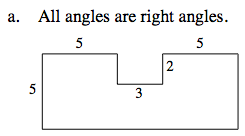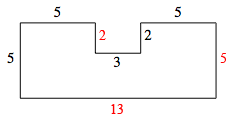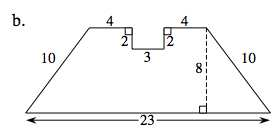### Home > MC2 > Chapter 6 > Lesson 6.2.1 > Problem6-81

6-81.Label the missing sides. Notice that the shape is a rectangle with a 2 by 3 section removed.To find the perimeter, add the value of each side. To find the area, multiply the base of the rectangle by the height and subtract the missing area.

Perimeter = 40 units
Area = 65 − 6 = 59 units2To find the perimeter, add the values of all the sides. To find the area, recall how to find the area of a trapezoid, then subtract the missing 2 by 3 section.

Area of a trapezoid.

$\text{Area}=\left(\frac{1}{2}\right)(\text{base}_1+\text{base}_2)(\text{height})$